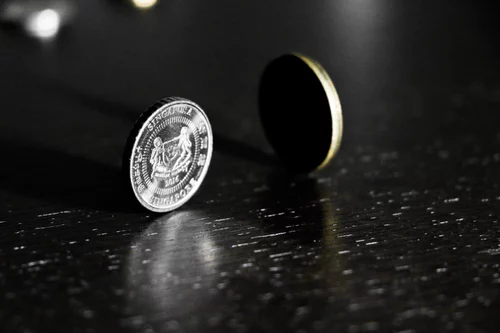# Experimental Probability or Relative Frequency

## Recording and describing the outcomes of experiments

When we do experiments we can calculate the experimental probability of something happening or not happening.

This is often referred to as relative frequency.

The relative frequency of something happening or the experimental probability is found by using this formula:

The number of outcomes / The number of trials

For example,

I might throw a coin and count how many times it lands on tails.To record my data I am going to use a simple table like this:

 Number of throw Outcome of event 1 Head 2 Tail 3 Head 4 Tail 5 Tail 6 Tail

From this table I can see that out of the 6 times I threw the coin it landed on a Head twice and a Tail 4 times.

Therefore I can say that the experimental probability of throwing a tail, according to my experiment is 4/6 or 2/3. This is different from the theoretical probability that would be 1/2 .

This is because my data comes from my experiment.

## Expected Outcomes

Predicting the number of outcomes

I can use my experimental probability to make further predictions. For example, from my experiment I found that there is a 2/3 chance of throwing a tail.

Therefore I can predict, that if I were to throw 60 coins, I would expect to get 40 Tails.

From an experiment, I know the probability of picking a number 5 from a bag and replacing it is 0.4. If I pick out numbers 10 more times how many 5’s would I expect to get?
4
In an experiment a dropped pin lands downwards 4 times out of 20 trials. What is the experimental probability of the pin landing downwards (give your answer as a decimal).
0.2
How can you improve the reliability of an experiment?
Your answer should include: Repeat / Re-do